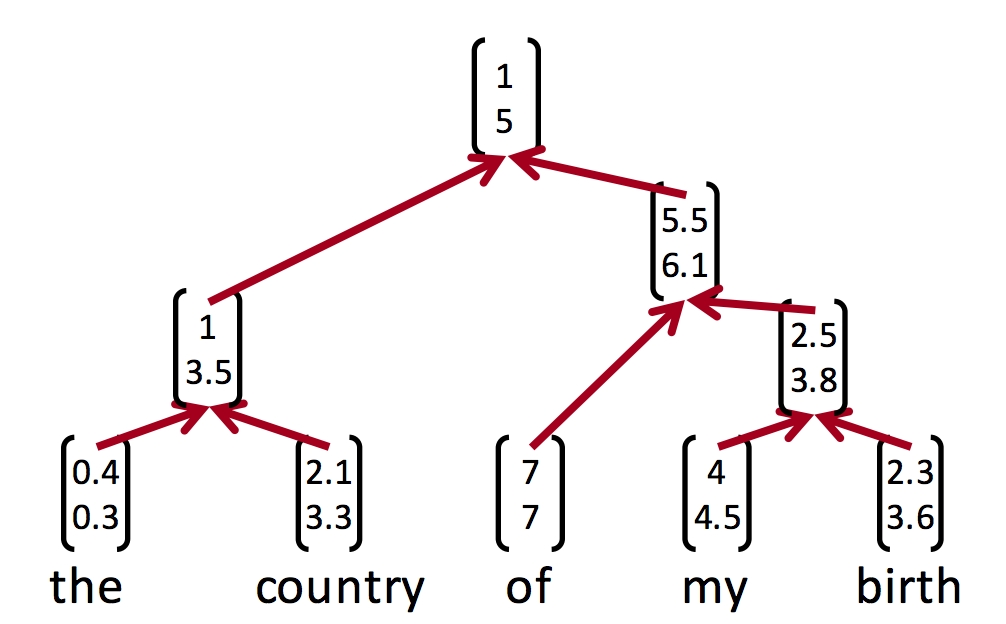2 views

I have a tree, specifically a parse tree with tags at the nodes and strings/words at the leaves. I want to pass this tree as input into a neural network all the while preserving its structure.

Current approach Assume we have some dictionary of words w1,w2.....wn Encode the words that appear in the parse tree as n-dimensional binary vectors with a 1 showing up in the ith spot whenever the word in the parse tree is wi

Now how about the tree structure? There are about 2^n possible parent tags for n words that appear at the leaves So we can't set a max length of input words and then just brute force enumerate all trees.

Right now all I can think of is to approximate the tree by choosing the direct parent of a leaf. This can be represented by a binary vector as well with dimension equal to a number of different types of tags - on the order of ~ 100 I suppose. My input is then two dimensional. The first is just the vector representation of a word and the second is the vector representation of its parent tag

Except this will lose a lot of the structure in the sentence. Is there a standard/better way of solving this problem?

by (33.1k points)

You can simply use a recursive neural network, which can be implemented using TensorFlow.

Check out this repository for an example implementation: https://github.com/erickrf/treernn

The following image might explain this method. The tree learns a representation of each leaf, and through the parents to finally construct the representation of the whole structure.